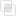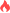python如何快速建立生成器

python生成器2021-09-11 09:42:042059浏览 · 0收藏 · 0评论1、用小括号包围数据的生成规则，创建一个生成器类型的对象。

```a=(x for x in range(10))
print(type(a))

#运行结果:
#<class 'generator'>```

2、函数+yield关键字创建法。需要将其写成多行函数，并通过调用该函数返回生成器对象。

```def fib():
n = 0
a,b = 0,1
while n<10:
n+=1
print(b,end=" ")
a,b=b,a+b
fib()

#运行结果:
#1 1 2 3 5 8 13 21 34 55

def fib():
n = 0
a,b = 0,1
while n<10:
n+=1
print(b,end=" ")
yield
a,b=b,a+b
a = fib()
print(type(a))

#运行结果:
#<class 'generator'>```python创建实例中类属性的变化python默认参数的使用注意python参数组合有什么使用规则python变长参数的使用注意python变长参数的使用场景python建立文本文件的两种途径python多行读写的操作Python修改matplotlib中的字体python如何解决黏包问题python链表的乘法问题python链表实现左移和右移Python绘图项目之海绵宝宝Python爬虫抓取名人名言网站python多线程的线程安全实现python爬虫的时候有哪些技巧可以借鉴？js事件委托如何理解27689

js中hasOwnProperty的属性用法27014